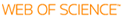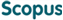### BROWSE

#### Related ResearcherHessari, Peyman
School of Natural Science
Research Interests
• Spectral Element Method
• Finite Element Method
• First Order System Least Squares Method
• Interface Problem
• Numerical Methods in Linear Algebra

First order system least squares method for the interface problem of the Stokes equations

Cited 0 times inCited 0 times inTitle
First order system least squares method for the interface problem of the Stokes equations
Author
Keywords
First order system least squares method; Interface problem; Spectral collocation method; Stokes equation
Issue Date
2014-08
Publisher
PERGAMON-ELSEVIER SCIENCE LTD
Citation
COMPUTERS & MATHEMATICS WITH APPLICATIONS, v.68, no.3, pp.309 - 324
Abstract
The first order system least squares method for the Stokes equation with discontinuous viscosity and singular force along the interface is proposed and analyzed. First, interface conditions are derived. By introducing a physical meaningful variable such as the velocity gradient, the Stokes equation transformed into a first order system of equations. Then the continuous and discrete norm least squares functionals using Legendre and Chebyshev weights for the first order system are defined. We showed that continuous and discrete homogeneous least squares functionals are equivalent to appropriate product norms. The spectral convergence of the proposed method is given. A numerical example is provided to support the method and its analysis.
URI
DOI
10.1016/j.camwa.2014.06.003
ISSN
0898-1221
Appears in Collections:
PHY_Journal Papers
Files in This Item: